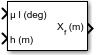Documentation

# LLA to ECEF Position

Calculate Earth-centered Earth-fixed (ECEF) position from geodetic latitude, longitude, and altitude above planetary ellipsoid

## Library

Utilities/Axes Transformations## Description

The LLA to ECEF Position block converts geodetic latitude $\left(\overline{\mu }\right)$, longitude $\left(\overline{\iota }\right)$, and altitude $\left(\overline{h}\right)$ above the planetary ellipsoid into a 3-by-1 vector of ECEF position $\left(\overline{p}\right)$. Latitude and longitude values can be any value. However, latitude values of +90 and -90 may return unexpected values because of singularity at the poles. The ECEF position is calculated from geocentric latitude at mean sea-level (λs) and longitude using:

`$\overline{p}=\left[\begin{array}{c}{\overline{p}}_{x}\\ {\overline{p}}_{y}\\ {\overline{p}}_{z}\end{array}\right]=\left[\begin{array}{c}{r}_{s}\mathrm{cos}{\lambda }_{s}\mathrm{cos}\iota +h\mathrm{cos}\mu \mathrm{cos}\iota \\ {r}_{s}\mathrm{cos}{\lambda }_{s}\mathrm{sin}\iota +h\mathrm{cos}\mu \mathrm{sin}\iota \\ {r}_{s}\mathrm{sin}{\lambda }_{s}+h\mathrm{sin}\mu \end{array}\right]$`

where geocentric latitude at mean sea-level and the radius at a surface point (rs) are defined by flattening $\left(\overline{f}\right)$, and equatorial radius $\left(\overline{R}\right)$ in the following relationships.

`$\begin{array}{l}{\lambda }_{s}=\text{atan}\left({\left(}^{1}\mathrm{tan}\mu \right)\\ \\ {r}_{s}=\sqrt{\frac{{R}^{2}}{1+\left(1/{\left(1-f\right)}^{2}-1\right){\mathrm{sin}}^{2}{\lambda }_{s}}}\end{array}$`

## Parameters

Units

Specifies the parameter and output units:

`Metric (MKS)`MetersMetersMeters
`English`FeetFeetFeet

This option is only available when Planet model is set to `Earth (WGS84)`.

Planet model

Specifies the planet model to use: `Custom` or ```Earth (WGS84)```.

Flattening

Specifies the flattening of the planet. This option is only available with Planet model set to `Custom`.

Specifies the radius of the planet at its equator. The units of the equatorial radius parameter should be the same as the units for altitude. This option is only available with Planet model set to `Custom`.

## Inputs and Outputs

InputDimension TypeDescription

First

2-by-1 vector Contains the geodetic latitude and longitude, in degrees.

Second

ScalarContains the altitude above the planetary ellipsoid.
OutputDimension TypeDescription

First

3-by-1 vectorContains the position in ECEF frame, in same units as altitude.

## Assumptions and Limitations

The planet is assumed to be ellipsoidal. To use a spherical planet, set the Flattening parameter to zero.

The implementation of the ECEF coordinate system assumes that the origin is at the center of the planet, the x-axis intersects the Greenwich meridian and the equator, the z-axis being the mean spin axis of the planet, positive to the north, and the y-axis completes the right-handed system.

## References

Stevens, B. L., and F. L. Lewis, Aircraft Control and Simulation, John Wiley & Sons, New York, 1992.

Zipfel, P. H., Modeling and Simulation of Aerospace Vehicle Dynamics, AIAA Education Series, Reston, Virginia, 2000.

“Atmospheric and Space Flight Vehicle Coordinate Systems,” ANSI/AIAA R-004-1992.

#### Introduced before R2006a

##### SupportGet trial now